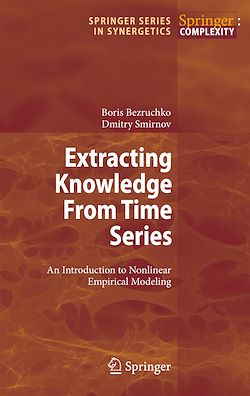# Extracting Knowledge From Time Series

-English

Description

Mathematical modelling is ubiquitous. Almost every book in exact science touches on mathematical models of a certain class of phenomena, on more or less speci?c approaches to construction and investigation of models, on their applications, etc. As many textbooks with similar titles, Part I of our book is devoted to general qu- tions of modelling. Part II re?ects our professional interests as physicists who spent much time to investigations in the ?eld of non-linear dynamics and mathematical modelling from discrete sequences of experimental measurements (time series). The latter direction of research is known for a long time as “system identi?cation” in the framework of mathematical statistics and automatic control theory. It has its roots in the problem of approximating experimental data points on a plane with a smooth curve. Currently, researchers aim at the description of complex behaviour (irregular, chaotic, non-stationary and noise-corrupted signals which are typical of real-world objects and phenomena) with relatively simple non-linear differential or difference model equations rather than with cumbersome explicit functions of time. In the second half of the twentieth century, it has become clear that such equations of a s- ?ciently low order can exhibit non-trivial solutions that promise suf?ciently simple modelling of complex processes; according to the concepts of non-linear dynamics, chaotic regimes can be demonstrated already by a third-order non-linear ordinary differential equation, while complex behaviour in a linear model can be induced either by random in?uence (noise) or by a very high order of equations.

Subjects

##### Classwork

Informations